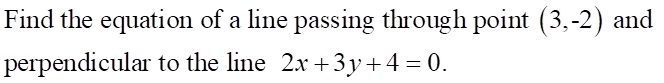# Find the equation of a line passing through point (3,-2) and perpendicular to the line 2x + 3y + 4-0.

Question

I need help with this question.help_outlineImage TranscriptioncloseFind the equation of a line passing through point (3,-2) and perpendicular to the line 2x + 3y + 4-0. fullscreen

Step 1

To find slope of the given line, we express it in a standard form, y = mx + c, we get:

Step 2

Comparing the equation obtained above with the standard form, we get:

Step 3

If m1 and m2 are the slopes of two lines that are perpendicular to each other, then we have, m1* m2 = -1. Here, let m1 be the slope obtained above, then m1 = -2/3. Solving for m2,...

Subscribe to bartleby learn and ask 30 homework questions each month. Subject matter experts are on standby 24/7 when you’re stuck and questions are typically answered in as fast as 30 minutes.*

*Response times vary by subject and question complexity. Median response time is 34 minutes and may be longer for new subjects.

Subscribe to bartleby learn and ask 30 homework questions each month. Subject matter experts are on standby 24/7 when you’re stuck and questions are typically answered in as fast as 30 minutes.*